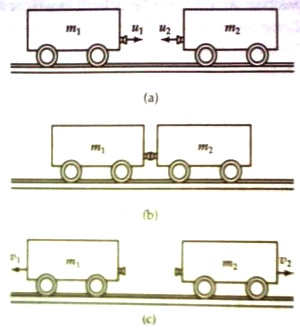Physics

# Application of Principle of Conservation of Mechanical EnergyApplication of principle of conservation of mechanical energy

In case of collision between two trains energy conversion of similar type takes place [Fig. a]. Before collision the trains only have kinetic energy. At the time of collision the two trains momentarily stop [Fig. b]. Then their kinetic energy becomes zero, i.e., kinetic energy changes to potential energy. If during collision the two trains act through a spring. Then the spring contracts i.e., work is done on the spring, consequently potential energy is stored in the spring. Next moment, the spring expands. Then the spring does work; as a result stored potential energy in the spring is used up. It is seen that the two trains moves away from each other (Fig- c). Now the two cars have only kinetic energy.Fig: transformation and conservation of mechanical energy

We can cite innumerable examples of conversion of mechanical energy— for example oscillation of simple pendulum and motion of a body in inclined surface. We can come to a very important conclusion from these examples. We know, energy can neither be created nor can be destroyed. So kinetic energy of the bodies, in these examples, are changed to potential energy only and vice versa; sum total of kinetic energy and potential energy is constant i.e., total mechanical energy is fixed. This is called the principle of conservation of mechanical energy. But frictional force always retards the motion of a body. So, a portion of total mechanical energy is spent or dissipated to overcome this resistance and is converted into heat energy.

In case of the above examples principle of conservation of mechanical energy is applicable. If there is no dissipated force and if the collision is perfectly elastic, then, total energy remains unchanged. Hence,

Kinetic energy before collision = stored potential energy = ½ m1v12 + ½ m2v22

When the magnitude of a quantity remains unchanged for any process, then that quantity is called conserved. So, total mechanical energy is conserved.